# MATLAB code starts here --------- clear T0=2; w0=2*pi/T0; f0=1/T0; Tmax=4; Nmax=15; %--- i=1; for t=-Tmax: .01:Tmax...

MATLAB

code starts here ---------

clear

T0=2;
w0=2*pi/T0;
f0=1/T0;
Tmax=4;
Nmax=15;

%---

i=1;
for t=-Tmax: .01:Tmax
T(i)=t;
if t>=(T0/2)
while (t>T0/2)
t=t-T0;
end
elseif t<=-(T0/2)
while (t<=-T0/2)
t=t+T0;
end
end
if abs(t)<=(T0/4)
y(i)=1;
else
y(i)=0;
end
i=i+1;
end
plot(T,y),grid, xlabel('Time (sec)'); title('y(t) square wave'); shg
disp('Hit return..');
pause
%---
a0=1/2;
F(1)=0; %dc freq
C(1)=a0;
for n=1:Nmax
a(n)=(2/(n*pi))*sin((n*pi)/2);
b(n)=0;
C(n+1)=sqrt(a(n)^2+b(n)^2);
F(n+1)=n*f0;
end
stem(F,abs,(C)), grid, title(['Line Spectrum: Harmonics = ' num2str(Nmax)]);
xlabel('Freq(Hz)'), ylabel('Cn'), shg
disp('Hit return...');
pause
%---
yest=a0*ones(1,length(T));
for n=1:Nmax
yest=yest+a(n)*cos(2*n*pi*T/T0)+b(n)*sin(2*n*pi*T/T0);
plot(T,y,T,yest),grid,
title(['Nmax = ' num2str(n)]), shg, pause(1)
end
code ends here ------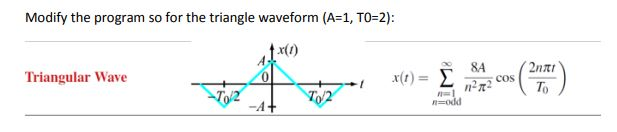Modify the program so for the triangle waveform (A-1, T0 2) Triangular Wave 8A Znat 5 COS が形 n=1

MATLAB code is given in bold letters.

clc;
close all;
clear all;
T0=2;
w0=2*pi/T0;
f0=1/T0;
Tmax=4;
Nmax=15;
i=1;
a0=0;
F(1)=0;
A = 1; T0 =2;
n = 1:2:15;
a= 8*A./(n*pi).^2;
b=0;
C=sqrt(a.^2+b^2);
F(n+1)=n*f0;
stem([0 n],[a0 abs(C)]), grid, title(['Line Spectrum: Harmonics = ' num2str(Nmax)]);
xlabel('k'), ylabel('Ck')
disp('Hit return...');
pause
%---
t = -4:0.01:4;

for k=1:length(t)

x(k) = a0+sum(C.*cos(2*pi*n*t(k)/T0));

end

figure;plot(t,x);xlabel('time');
ylabel('Amplitude');title('x(t)');grid on;
title('Fourier series approximation with N = 15');
ylim([-1.5 1.5]);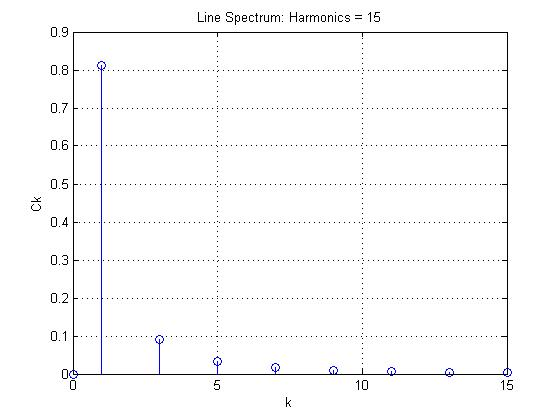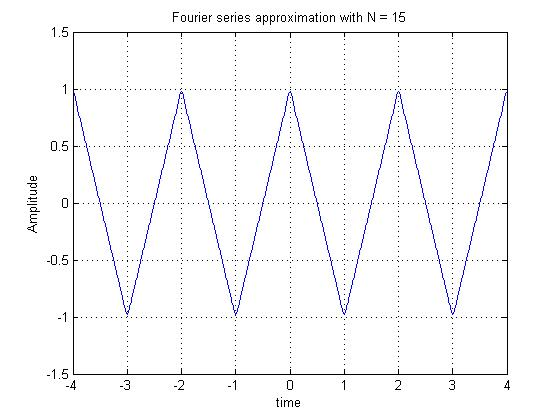#### Earn Coin

Coins can be redeemed for fabulous gifts.

Similar Homework Help Questions
• ### You are given a finite step function xt=-1  0<t<4 1  4<t<8.           Hand calculate the FS coefficients of...

You are given a finite step function xt=-1  0<t<4 1  4<t<8.           Hand calculate the FS coefficients of x(t) by assuming half- range expansion, for each case below. Modify the code below to approximate x(t) by cosine series only (This is even-half range expansion). Modify the below code and plot the approximation showing its steps changing by included number of FS terms in the approximation. Modify the code below to approximate x(t) by sine series only (This is odd-half range expansion).. Modify...

• ### % THIS PROGRAM IS A MATLAB PROGRAM THAT DESIGNS AND THEN COMPUTES THE % ANTENNA RADIATION...

% THIS PROGRAM IS A MATLAB PROGRAM THAT DESIGNS AND THEN COMPUTES THE % ANTENNA RADIATION CHARACTERISTICS OF: % % I. RECTANGULAR % II. CIRCULAR % % MICROSTRIP PATCH ANTENNAS BASED ON THE CAVITY MODEL AND DOMINANT % MODE OPERATION FOR EACH. THAT IS: % % A. TM(010) MODE FOR THE RECTANGULAR PATCH % B. TM(011) MODE FOR THE CIRCULAR PATCH % % ** INPUT PARAMETERS % 1. FREQ = RESONANT FREQUENCY (in GHz) % 2. EPSR = DIELECTRIC CONSTANT...

• ### please help me with this MATLAB CODE and explain to me what each line does and...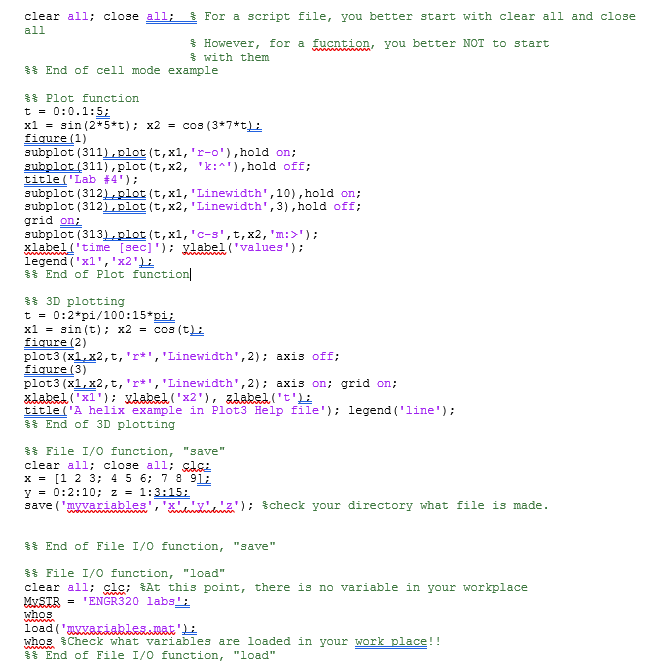please help me with this MATLAB CODE and explain to me what each line does and what is used for? leave your comments as words, not as pictures. ..................................................................................................................................................................... clear all; close all; % For a script file, you better start with clear all and close all                        % However, for a fucntion, you better NOT to start                        % with them %% End of cell mode example %% Plot function t = 0:0.1:5; x1 = sin(2*5*t); x2 = cos(3*7*t);...

• ### This is a MATLAB Question. Below is my base code for a Fourier Series of a...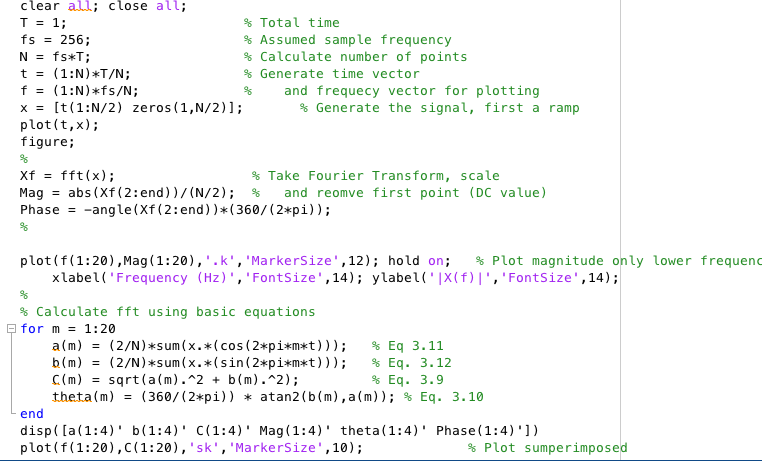This is a MATLAB Question. Below is my base code for a Fourier Series of a half triangle wave. So I am being asked to isolate the first 8 components so that only those first 8 components of the half triangle function are calculated by MATLAB fft function, and then afterwards I am asked to do the same thing but with the first 20 components rather than the first 8. How do I isolate the first x number of components...

• ### I have all of the answers to this can someone just actually explain this matlab code and the results to me so i can get a better understanding? b) (c) and (d) %% Matlab code %% clc; close all; clear...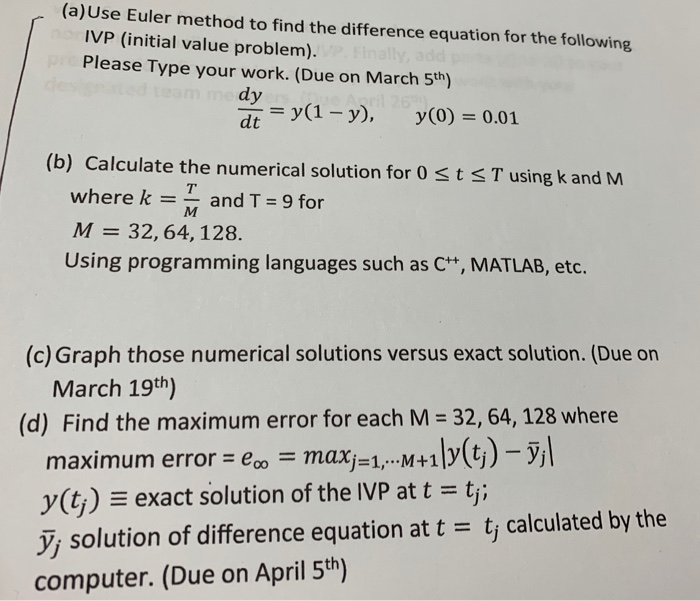I have all of the answers to this can someone just actually explain this matlab code and the results to me so i can get a better understanding? b) (c) and (d) %% Matlab code %% clc; close all; clear all; format long; f=@(t,y)y*(1-y); y(1)=0.01; %%%% Exact solution [t1 y1]=ode45(f,[0 9],y(1)); figure; plot(t1,y1,'*'); hold on % Eular therom M=[32 64 128]; T=9; fprintf(' M Max error \n' ); for n=1:length(M) k=T/M(n); t=0:k:T; for h=1:length(t)-1 y(h+1)=y(h)+k*f(t(h),y(h)); end plot(t,y); hold on %%%...

• ### the code in the photo for this I.V.P dy/dx= x+y. y(0)=1 i need the two in the photo thank you New folder Bookmark...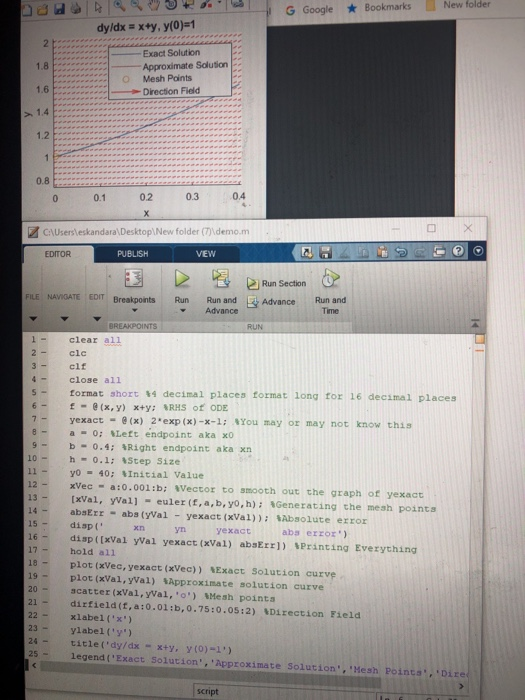the code in the photo for this I.V.P dy/dx= x+y. y(0)=1 i need the two in the photo thank you New folder Bookmarks G Google dy/dx x+y, y(0)=1 2 h Exact Solution 1.8 Approximate Solution Mesh Points 1.6 -Direction Fied 1.4 1.2 1 0.8 04 0.2 0.3 0.1 0 X CAUsersleskandara\Desktop\New folder emo.m EDITOR PUBLISH VEW Run Section FILE NAVIGATE EDIT Breakpoints Run Run and FL Advance Run and Advance Time BREAKPOINTS RUN 1 - clear all 2 clc 3-...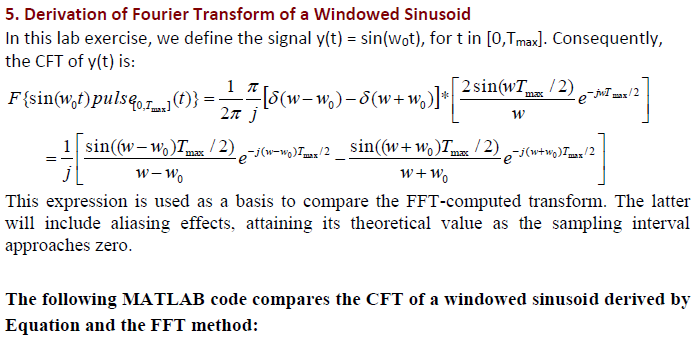Can you please help me answer Task 2.b? Please show all work. fs=44100; no_pts=8192; t=([0:no_pts-1]')/fs; y1=sin(2*pi*1000*t); figure; plot(t,y1); xlabel('t (second)') ylabel('y(t)') axis([0,.004,-1.2,1.2]) % constrain axis so you can actually see the wave sound(y1,fs); % play sound using windows driver. %% % Check the frequency domain signal. fr is the frequency vector and f1 is the magnitude of F{y1}. fr=([0:no_pts-1]')/no_pts*fs; %in Hz fr=fr(1:no_pts/2); % single-sided spectrum f1=abs(fft(y1)); % compute fft f1=f1(1:no_pts/2)/fs; %% % F is the continuous time Fourier. (See derivation...

• ### Hi I need help understanding this matlab code. Can you add comments or at least state what the lines in this code are doing. Thanks! close all; clear all;clc; t=1:0.025:5; desired=5*sin(2*3.*t); noi...

Hi I need help understanding this matlab code. Can you add comments or at least state what the lines in this code are doing. Thanks! close all; clear all;clc; t=1:0.025:5; desired=5*sin(2*3.*t); noise=5*sin(2*50*3.*t); refer=5*sin(2*50*3.*t+ 3/20); primary=desired+noise; subplot(4,1,1); plot(t,desired); ylabel('desired'); subplot(4,1,2); plot(t,refer); ylabel('refer'); subplot(4,1,3); plot(t,primary); ylabel('primary'); order=2; mu=0.005; n=length(primary) delayed=zeros(1,order); adap=zeros(1,order); cancelled=zeros(1,n); for k=1:n, delayed(1)=refer(k); y=delayed*adap'; cancelled(k)=primary(k)-y; adap = adap + 2*mu*cancelled(k) .* delayed; delayed(2:order)=delayed(1:order-1); end subplot(4,1,4); plot(t,cancelled); ylabel('cancelled');

• ### Solve using Matlab Use the forward Euler method, Vi+,-Vi+(4+1-tinti ,Vi) for i= 0,1,2, , taking yo y(to) to be the initial condition, to approximate the solution at t-2 of the IVP y'=y-t2 + 1, 0-...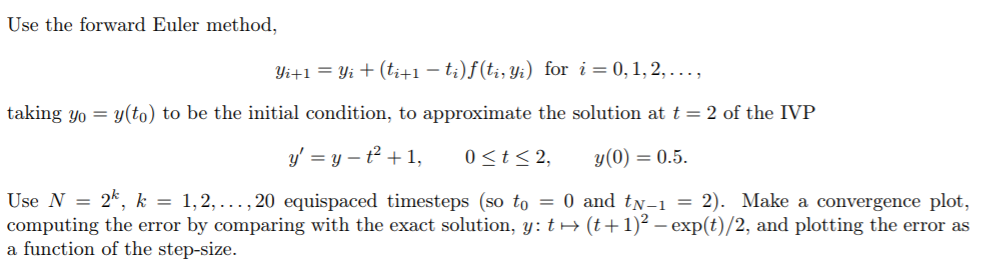Solve using Matlab Use the forward Euler method, Vi+,-Vi+(4+1-tinti ,Vi) for i= 0,1,2, , taking yo y(to) to be the initial condition, to approximate the solution at t-2 of the IVP y'=y-t2 + 1, 0-t-2, y(0) = 0.5. Use N = 2k, k = 1, 2, , 20 equispaced time steps (so to = 0 and tN-1 = 2). Make a convergence plot, computing the error by comparing with the exact solution, y: t1)2 -exp(t)/2, and plotting the error as...

Free Homework Help App